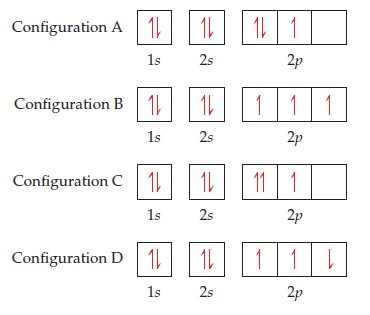# Problem: Four possible electron configurations for a nitrogen atom are shown below, but only one schematic represents the correct configuration for a nitrogen atom in its ground state. Which one is the correct electron configuration? Which one is the correct electron configuration?

⚠️Our tutors found the solution shown to be helpful for the problem you're searching for. We don't have the exact solution yet.

###### Problem Details

Four possible electron configurations for a nitrogen atom are shown below, but only one schematic represents the correct configuration for a nitrogen atom in its ground state. Which one is the correct electron configuration?Which one is the correct electron configuration?